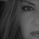# Indicators: Rainbow Charts Oscillator, Binary Wave and MAs

10944 views
Rainbow Charts , by Mel Widner, is a trend detector. It uses recursively smoothed MAs (remember, this idea was proposed back in 1997 -- it was certainly cool back then!) and also builds an oscillator out of the MAs. Oscillator bands indicate the stability range.

I have also included a simple binary wave based on whether all the MAs are in an upward slope or not. If you see any value above 0.5 there, the trend is definitely up (all MAs pointing up).

Here's my complete list of indicators (With these 3, the total count should be above 100 now...will update the list later today)
```//
// @author LazyBear
//
// If you use this code in its original/modified form, do drop me a note.
//
study("Rainbow Charts Oscillator [LazyBear]", shorttitle="RCO_LB")
sma2=sma(close,2)
dsma2=sma(sma2,2)
tsma2=sma(dsma2,2)
qsma2=sma(tsma2,2)
psma2=sma(qsma2,2)
ssma2=sma(psma2,2)
s2sma2=sma(ssma2,2)
osma2=sma(s2sma2,2)
o2sma2=sma(osma2,2)
desma2=sma(o2sma2,2)

rmax=max(sma2,max(dsma2,max(tsma2,max(qsma2,max(psma2,max(ssma2,max(s2sma2,max(osma2,max(o2sma2,desma2)))))))))
rmin=min(sma2,min(dsma2,min(tsma2,min(qsma2,min(psma2,min(ssma2,min(s2sma2,min(osma2,min(o2sma2,desma2)))))))))
rosc=100*(close-((sma2+dsma2+tsma2+qsma2+psma2+ssma2+s2sma2+osma2+o2sma2+desma2)/10))/(highest(close,10)-lowest(close,10))
rbl=-100*(rmax-rmin)/(highest(close,10)-lowest(close,10))
rbu=-rbl //100*(rmax-rmin)/(highest(close,10)-lowest(close,10))
ml=plot(0)
ll=plot(rbl, color=gray)
ul=plot(rbu, color=gray)
plot(rosc, color=rosc>=0?green:red, linewidth=3, style=histogram)

fill(ll, ml, red)
fill(ml, ul, green)```
List of my free indicators: http://bit.ly/1LQaPK8
List of my indicators at Appstore: http://blog.tradingview.com/?p=970